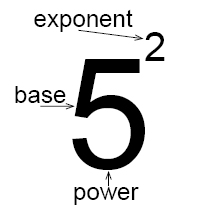# Powers and exponents

We know how to calculate the expression 5 x 5. This expression can be written in a shorter way using something called exponents.

$5\cdot 5=5^{2}$

An expression that represents repeated multiplication of the same factor is called a power.

The number 5 is called the base, and the number 2 is called the exponent. The exponent corresponds to the number of times the base is used as a factor.31 3 to the first power 3 42 4 to the second power or 4 squared 4 ∙ 4 53 5 to the third power or 5 cubed 5 ∙ 5 ∙ 5 26 2 to the power of six 2 ∙ 2 ∙ 2 ∙ 2 ∙ 2 ∙ 2

Example

Write these multiplications like exponents

$5\cdot 5\cdot 5=5^{3}$

$4\cdot 4\cdot 4\cdot 4\cdot 4=4^{5}$

$3\cdot 3\cdot 3\cdot 3=3^{4}$

### Multiplication

If two powers have the same base then we can multiply the powers. When we multiply two powers we add their exponents.

The rule:

$x^{a}\cdot x^{b}=x^{a+b}$

Example

$4^{2}\cdot 4^{5}=\left ( 4\cdot 4 \right )\cdot \left ( 4\cdot 4\cdot 4\cdot 4\cdot 4 \right )=4^{7}=4^{2+5}$

### Division

If two powers have the same base then we can divide the powers. When we divide powers we subtract their exponents.

The rule:

$\frac{x^{a}}{ x^{b}}=x^{a-b}$

Example

$\frac{4^{2}}{ 4^{5}}=\frac{{\color{red} {\not}{4}}\cdot {\color{red} {\not}{4}}}{{\color{red} {\not}{4}}\cdot {\color{red} {\not}{4}}\cdot 4\cdot 4\cdot 4}=\frac{1}{4^{3}}=4^{-3}=4^{2-5}$

A negative exponent is the same as the reciprocal of the positive exponent.

$x^{-a}=\frac{1}{x^{a}}$

Example

$2^{-3}=\frac{1}{2^{3}}$

When you raise a product to a power you raise each factor with a power

$(x\cdot y)^{a}=x^{a}\cdot y^{a}$

Example

$(2x)^{4}=2^{4}\cdot x^{4}=16x^{4}$

The rule for the power of a power and the power of a product can be combined into the following rule:

$(x^{a}\cdot y^{b})^{z}=x^{a\cdot z}\cdot y^{b\cdot z}$

Example

$(x^{3}\cdot y^{4})^{2}=x^{3\cdot 2}\cdot y^{4\cdot 2}=x^{6}\cdot y^{8}$

## Video lessons

Rewrite the expressions

$2\cdot 2\cdot 2$

$x\cdot x\cdot x\cdot x\cdot x$

$3^{4}$

$x^{3}$

Simplify the expression

$\left ( x^{2}\cdot y^{3}\cdot z^{5} \right )^{3}$